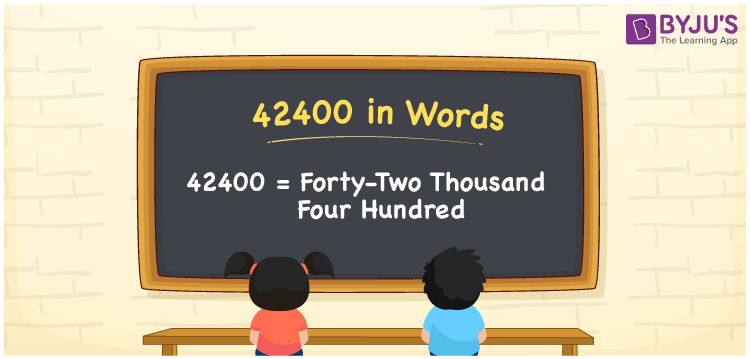# 42400 in words

42400 in words is written as Forty Two Thousand Four Hundred. In 42400, 4 has a place value of ten thousand, 2 is in the place value of thousand and next 4 is in the place value of hundred. The article on Place Value gives more information. The number 42400 is used in expressions that relate to money, distance, social media views, and many more. For example, “42.2 Hectare is nothing but Forty Two Thousand four Hundred square meters”. Another example, “Today’s total pandemic cases rose to Forty Two Thousand Four Hundred”.

 42400 in words Forty Two Thousand Four Hundred Forty Two Thousand Four Hundred in Numbers 42400

## 42400 in English Words## How to Write 42400 in Words?

We can convert 42400 to words using a place value chart. The number 42400 has 5 digits, so let’s make a chart that shows the place value up to 5 digits.

 Ten thousand Thousands Hundreds Tens Ones 4 2 4 0 0

Thus, we can write the expanded form as:

4 × Ten thousand + 2 × Thousand + 4 × Hundred + 0 × Ten + 0 × One

= 4 × 10000 + 2 × 1000 + 4 × 100 + 0 × 10 + 0 × 1

= 42400.

= Forty Two Thousand Four Hundred.

42400 is the natural number that is succeeded by 42399 and preceded by 42401.

42400 in words – Forty Two Thousand Four Hundred.

Is 42400 an odd number? – No.

Is 42400 an even number? – Yes.

Is 42400 a perfect square number? – No.

Is 42400 a perfect cube number? – No.

Is 42400 a prime number? – No.

Is 42400 a composite number? – Yes.

## Solved Example

1. Write the number 42400 in expanded form

Solution: 4 x 10000 + 2 x 1000 + 4 x 100 + 0 x 10 + 0 x 1

Or Just 4 x 10000 + 2 x 1000 + 4 x 100

We can write 42400 = 40000 + 2000 + 400 + 0 + 0

= 4 x 10000 + 2 x 1000 + 4 x 100 + 0 x 10 + 0 x 1.

## Frequently Asked Questions on 42400 in words

Q1

### How to write the number 42400 in words?

42400 in words is written as Forty Two Thousand Four Hundred.
Q2

### State whether True or False. 42400 is divisible by 3?

False. 42400 is not divisible by 3.
Q3

### Is 42400 divisible by 10?

Yes. 42400 is divisible by 10.